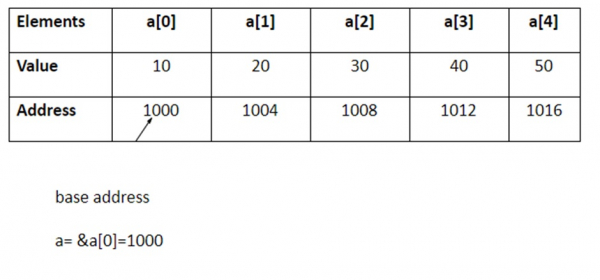# Explain the concepts of Pointers and arrays in C language

## Pointers and arrays

Continuous memory locations are allocated for all the elements of the array by the compiler.

The base address is the location of the first element in the array.

For example, int a  = {10, 20,30,40,50};

The five elements are stored as follows −If ‘p’ is declared as integer pointer, then the array ‘a’ can be pointed by the following assignment −

p=a
or
p=&a;

Each value of ‘a’ is accessed by using p++ to move from one element to another. When a pointer is incremented, its value is increased by the size of the datatype that it points to. This length is called the “scale factor”.

The relationship between pointer p and variable a is shown below −

P   = &a = 1000
P+1 = &a = 1004
P+2 = &a = 1008
P+3 = &a = 1012
P+4 = &a = 1016

Address of an element is calculated using its index and the scale factor of the datatype.

## Example

=1000+(3*4)

=1000+12

=1012

*(p+3) gives the value of a
a[i] = *(p+i)

## Program

#include<stdio.h>
main (){
int a;
int *p,i;
clrscr ();
printf (”Enter 5 lements”);
for (i=0; i<5; i++)
scanf (“%d”, &a[i]);
p = &a;
printf (“Elements of the array are”);
for (i=0; i<5; i++)
printf(“%d”, *(p+i));
getch();
}

## Output

Enter 5 elements : 10 20 30 40 50
Elements of the array are : 10 20 30 40 50